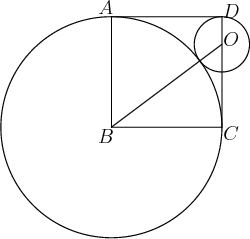# A surprising answerIn the diagram, the circle centered at $B$ and the circle centered at $O$ are tangent inside square $ABCD$. If $\cos{\angle{OBC}}=\frac{a}{b}$ for coprime positive integers $a$ and $b$, then what is $a+b$?

×